﻿ Initial Value Technique for Singular Perturbation Boundary Value Problems

### Initial Value Technique for Singular Perturbation Boundary Value ProblemsOPEN ACCESSPEER-REVIEWED

## Initial Value Technique for Singular Perturbation Boundary Value Problems

Hradyesh Kumar MishraDepartment of Mathematics, Jaypee University of Engineering & Technology, Raghogarh, Guna(M.P) India

### Abstract

In this paper, an initial-value approach is presented for solving singularly perturbed two-point boundary value problems with the boundary layer at left end point. The given singularly perturbed two-point boundary value problems replaced by first-order problem. This first-order problem is solved by classical Runge- Kutta method. Numerical examples have been solved to demonstrate the applicability of the method. Graphs are shown in support of results.

### At a glance: Figures

12
Prev Next

• Mishra, Hradyesh Kumar. "Initial Value Technique for Singular Perturbation Boundary Value Problems." American Journal of Numerical Analysis 2.2 (2014): 49-54.
• Mishra, H. K. (2014). Initial Value Technique for Singular Perturbation Boundary Value Problems. American Journal of Numerical Analysis, 2(2), 49-54.
• Mishra, Hradyesh Kumar. "Initial Value Technique for Singular Perturbation Boundary Value Problems." American Journal of Numerical Analysis 2, no. 2 (2014): 49-54.

 Import into BibTeX Import into EndNote Import into RefMan Import into RefWorks

### 1. Introduction

The numerical solution of singular perturbation problems is currently a field in which active research is going on. Singular perturbation problems are of common occurrence in fluid mechanics and other branches of applied mathematics. For detailed analytical discussion on singular perturbation problems, one can refer to Bender and Orsazag , Kevorkian and Cole , Nayfeh [12, 13], O’s Mally . More recently, a non asymptotic method also called the boundary value technique has been introduced by Robert  to solve certain classes of singular perturbation problems. He also discussed the analytical and approximate solutions of several numerical examples. The concept of replacing singularly perturbed two-point boundary value problem by initial-value technique is presented by many authors; see for examples (cf. [2, 3, 5, 6, 8, 9, 10, 11, 16, 17]).

In this paper, we present an approximate method for the numerical solution of a class of singularly perturbed two-point boundary value problems with the boundary layer at left end point. The original problem is replaced by an asymptotically equivalent first-order problem which is solved by using boundary condition. Numerical examples have been solved to demonstrate the applicability of the method.

### 2. Description of the Method

We consider a class of singular perturbation problems of the form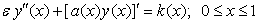(1)

with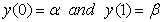(2)

where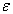is a small positive parameter (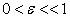);are given constants; a(x) and k(x) are assumed to be sufficiently continuously differentiable function in [0, 1]. Furthermore, we assume that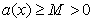through out the interval [0, 1], where M is some positive constant. This assumption merely implies that the boundary layer will be in the neighborhood of x=0. Now, we replace the original second-order problem by an asymptotically equivalent first-order problem as follows: By integrating Eq. (1), we obtain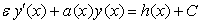(3)

where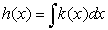and C is a constant to be determined. Settingin (3) and using boundary condition,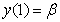; i.e.Or(4)

This value of C ensures that the solution of the reduced problem of Eq. (1) satisfies the reduced equation of the Eq. (3).

Now, we adjoin the conditionto Eq. (3) to obtain a first-order problem(5)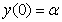(6)

where the constant C is given by Eq. (4). Thus, we have replaced the original second-order problem (1)-(2) with the asymptotically equivalent first-order problem (5)-(6). Now we solve Eq. (5) using Runge Kutta method. In fact, any standard analytically or numerical method can be used.

### 3. Numerical Examples

To demonstrate the applicability of the method, we will discuss two examples; a homogeneous singular perturbation problem (SPP), a non-homogeneous SPP. These examples have been chosen because either analytical or approximate solutions are available for comparison.

Example 3.1. Consider the following homogeneous SPP from Kevorkian and Cole , p.33, Eqs. (2.3.26) and (2.3.27) with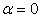],(7)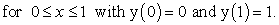(8)

The exact solution is given by(9)

By integrating Eq. (7), we get(10)

The constant C is determined by using Eq. (4), as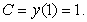(11)

Therefore,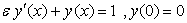(12)

On solving Eq. (12), we get approximate value of y(x).

The computational results are presented in Table 1 and Table 2 forand, respectively.

#### Table 2. Numerical results of Example 3.1 with ε=10-4, h=10-4

Example 3.2. Consider the following non-homogeneous SPP from fluid dynamics for fluid of small viscosity, Reinhardt [, Example2],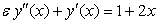(13)(14)

The exact solution is given by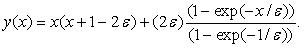(15)

By integrating Eq. (13), we get(16)

The constant C is determined by using Eq. (4), as(17)

Therefore,(18)

On solving Eq. (18), we get approximate value of y(x).

The computational results are presented in Table 3 and Table 4 for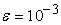and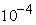, respectively.

### 4. General Problems

Here, we consider a more general class of problems of the form(19)(20)

whereis a small positive parameter;are given constants: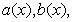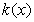are assumed to be sufficiently continuously differentiable functions in [0, 1]; andthroughout the interval [0, 1], where M is some positive constant.

#### Table 4. Numerical results of Example 3.2 with ε=10-4, h=10-4

It is clear that there will be difficulty in applying the integration process due to the presence of the term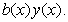To overcome this difficulty, we first modify

Eq. (19) and then apply our method.

Let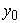be the solution of the reduced problem of (19)-(20); that is,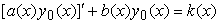(21)

with(22)

Now set up the approximate equation to the given equation (19) as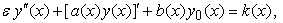(23)

where we have simply replaced the-term by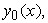the solution of the reduced problem (21)-(22). Then, we rewrite Eq. (23) in the form of Eq. (1) as(24)

where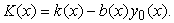Finally, we apply our method, from Eq. (1) to Eq. (6), to the modified problem (24).

Example 4.1. Consider the following singular perturbation problem from Bender and Orszag [, p. 480, problem 9.17 with]:(25)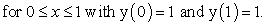(26)

The exact solution is given by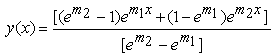(27)

whereand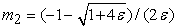.

From the previous step, we get an approximate equation to (25) as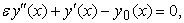(28)

Where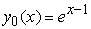is the solution of the reduced problem of (25)-(26);

i.e.(29)

with(30)

Then rewrite the Eq. (28) in the form of (1) as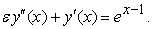(31)

By integrating Eq. (31), we get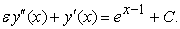(32)

The constant C is determined by using Eq. (4), as(33)

Therefore,(34)

On solving Eq. (34), we get approximate value of y(x).

The computational results are presented in Tables 5 and 6 forand, respectively.

### 5. Discussion and Conclusions

We have presented an initial-value approach for solving singularly perturbed two-point boundary value problems. In general, the numerical solution of a boundary value problem will be more difficult matter than the numerical solution of the corresponding initial-value problems. Hence, we always prefer to convert the second-order problem into first-order problems. Here, the given singularly perturbed two-point boundary value problem is replaced by first-order problem. This first-order problem is solved by classical Runge-Kutta method. We have tabulated the numerical results obtained by present method as well as the exact solution. Graphs are plotted for the tabulated results.

### References

  C.M. Bender, S.A. Orszag, Advanced Mathematical Methods for Scientists and Engineers, McGraw-Hill, New York, (1978).In article  M.K. Kadalbajoo, Y.N. Reddy, Initial-value technique for a class of non-linear singular perturbation problems, Journal of Optimization Theory and Applications, 53, (1987), 395-406.In article CrossRef  M.K. Kadalbajoo, Y.N. Reddy, An approximate method for solving a class of singular perturbation problems, Journal of Mathematical Analysis and Applications, 133, (1988), 306-323.In article CrossRef  J. Kevorkian, J.D. Cole, Perturbation Methods in Applied Mathematics, Springer- Verlag, New York, (1981).In article CrossRef  M.Kumar, P.singh, H.K.Mishra, An initial-value technique for singularly perturbed boundary value problems via cubic spline, International journal of Computational methods in Engineering Science and Mechanics 6(2007), 1-9.In article  M.Kumar, P.Singh, H.K.Mishra, Numerical treatment of singularly perturbed Two-point boundary value problems using initial value method, Journal of Applied Mathematics and Computation, 29(2009), 229-246.In article CrossRef  R.E.O’ Malley, Introduction to Singular Perturbations, Academic Press, New York, (1974).In article  Jia-qi Mo, Wan-tao Lin, The Nonlinear Singularly Perturbed Initial Boundary Value Problems of Non-local Reaction Diffusion Systems, Acta Mathematicae Applicatae Sinica, English Series. 22 (2006), 277-286.In article  S. Natesan, N.Ramanujam, Initial-value technique for singularly- perturbed turning point problem exhibiting twin boundary layers, Journal of Optimization Theory and Applications, 99 (1998) 37-52.In article CrossRef  S. Natesan, N.Ramanujam, Initial-value technique for singularly- perturbed BVPs for second order ordinary differential equations arising in chemical reactor theory, Journal of Optimization Theory and Applications, 97 (1998), 455-470.In article CrossRef  S. Natesan and N. Ramanujam, Shooting method” for the solution of singularly perturbed two-point boundary-value problems having less severe boundary layer Applied Mathematics and Computation, 133(2002), 623-641.In article CrossRef  A.H. Nayfeh, Introduction to Perturbation techniques, Wiley, New York, (1981).In article  A.H. Nayfeh, Perturbation Methods, Wiley, New York, (1979).In article  H.J. Reinhardt, Singular perturbations of difference methods for linear ordinary differential equations, Applicable Analysis, 10 (1980), 53-70.In article CrossRef  S.M. Roberts, A boundary-value technique for singular perturbation problems, Journal of Mathematical Analysis and Applications, 87 (1982) 489-503.In article CrossRef  T.Valanarasu, N.Ramanjam, Asymptotic initial-value method for singularly perturbed boundary value problems for second order ordinary differential equations, Journal of Optimization Theory and Applications, 116 (2003), 167-182.In article CrossRef  J. Vigo-Aguiar and S. Natesan, An efficient numerical method for singular perturbation problems, Journal of Computational and Applied Mathematics, 192 (2006)132-141.In article CrossRef